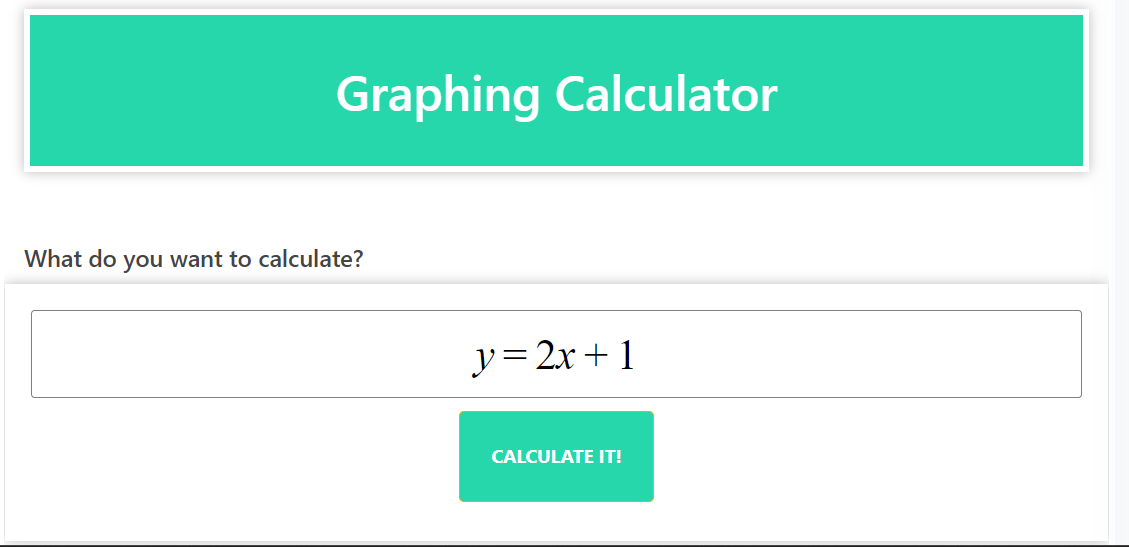# Graphing Calculator – Your Powerful Math Solver for Visualizing Functions

### Introduction:

Looking for a versatile tool for visualizing mathematical functions and equations? Our graphing calculator is here to help you. This advanced math solver allows you to plot graphs, explore functions, and gain a deeper understanding of mathematical concepts through visual representation.

### Explanation:

The Graphing Calculator is a valuable tool that helps you visualize functions and equations by plotting them on a coordinate plane. It simplifies the process of graphing complex functions and allows you to analyze their behavior, identify key points, and explore relationships between variables.

### Function Plotting:

The calculator enables you to input mathematical functions, such as linear, quadratic, trigonometric, exponential and logarithmic functions. It then plots the corresponding graph, allowing you to observe the shape, intercept, slope, and other important characteristics of the function.

### Multiple Graphs:

Our calculator supports plotting multiple functions on the same graph, which is especially useful for comparing different functions or analyzing their intersections and relationships. This feature facilitates the exploration of mathematical concepts and helps you gain insight into complex equations.

### Customization Options:

You can customize the appearance of the graph by adjusting the axes, gridlines, colors, and labels. This flexibility allows you to tailor the graph to your specific needs and preferences, enhancing your ability to effectively interpret and present mathematical data.

### Zooming and Panning:

The calculator allows you to zoom in or out on specific areas of the graph or pan in the coordinate plane. These interactive features enable detailed analysis of specific areas or focus on particular points, increasing your understanding of the function’s behavior.

### Solving Equations:

In addition to graphing functions, our calculator can also solve equations numerically. By inputting an equation, you can find points of intersection with the x-axis or other functions, facilitating the solution of equations and systems of equations.

Using our graphing calculator provides a visual representation of mathematical operations, making complex concepts more accessible and understandable. It promotes a deeper understanding of mathematical relationships and facilitates the analysis of functions in a variety of fields, including algebra, calculus, and statistics. Graphing Calculator (Maths solver)

### conclusion:

Extend your mathematical exploration and understanding with our graphing calculator. This powerful math solver enables you to plot functions, analyze their behavior, and visualize mathematical concepts. Experience the convenience and insight offered by our graphing calculator today, and uncover the beauty of mathematical functions through visual representation. Graphing Calculator (Maths solver)